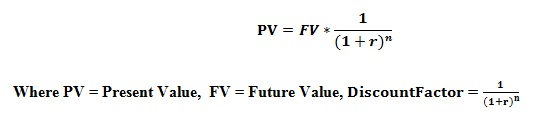# How to Calculate Present Value

The present value of future cash flows can be used to identify the amount that would be necessary to invest at present in order to generate a specific amount of income in the future. The process of calculating this amount is known as discounting, and the interest rate that is used for the calculation is known as the discount rate. To calculate present value of the future cash flows, the following formula can be used.For example, in order to get \$100 in one year’s time at 10% discount rate, the present value that needs to be invested can be calculated as follows:The present value factor is primarily based on the concept of the time value of money. It means that the value of money depreciates with time. In other words, \$100 at present is having a higher value than in another ten years’ time. Therefore, it is worth to reinvest the money received as an income at present in order to earn an additional amount.

## Factors affecting NPV

In general, there are three main factors that have an impact on the calculations of NPV as stated below:

• The amount of the cash flow

There is a relationship between the present cash flow and the future cash flow. The amount of the future cash flow depends on the amount in which the investor expects to invest at present. In order to derive a larger income in the future, the investor needs to invest a higher amount for the implementation of the project.

• The level of risk associated with the project

In the rapid changing, competitive business environment investing a huge amount for a new project is risky. The level of risk depends on the amount in which the investor is expected to invest on the project. The level of the risk can be calculated in comparison of the rate of return that can be generated by investing in another project having a similar type of risk.

• The time period of the investment

If the investment is for a longer time period, it will lower the value of cash flow. Instead of the long term investment, there are two options that can be considered as stated below:

a. If this money has been invested in some other project, the investor will be able to earn an annual interest, without waiting for a longer time period.

b. If the time period is so long, there is more and more risk about the ability to earn the expected income and cover up all the costs incurred as the money value also depreciates with time.